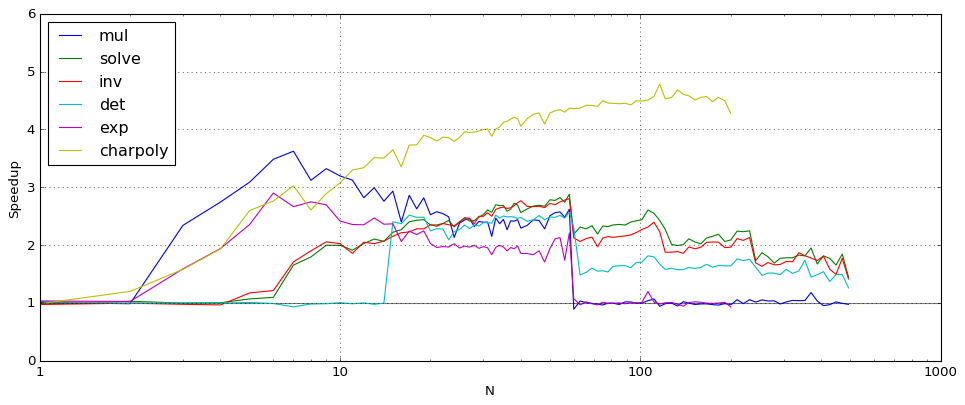# Accelerated arithmetic in Arb 2.15

September 18, 2018

I have just issued version 2.15 of Arb. As usual, downloads are available via GitHub.

The big news in Arb 2.15 is that polynomial and matrix arithmetic is significantly faster in the basecase regimes. The speedup is typically about 2x for polynomials and matrices of size 10 to 30 when the precision is 1-2 words (up to 128 bits), with varying levels of improvement in other ranges. For certain operations, more than a 4x speedup is possible. The improvement is due to use of fast dot product evaluation in combination with more accurate cutoffs between different algorithms.

Arb 2.15 adds the functions arb_dot and acb_dot for computing dot products $\sum_{k=1}^N a_k b_k$. The normal way to compute a dot product until now was to do fused multiply-adds with arb_addmul / acb_addmul in a loop. However, each such multiply-add has to produce fully normalized arb_t or acb_t output. The main idea behind the new dot product functions is to treat the whole dot product as an atomic operation and avoid normalization and bookkeeping overhead for each partial sum. In addition to this, the new dot product functions inspect the error bounds for all entries in the vectors and for each term $a_k b_k$ only compute the leading bits that actually contribute to the final result, saving even more time when the terms vary in magnitude or have large radii.

There are also new functions arb_approx_dot and acb_approx_dot which compute floating-point dot products without error bounds, useful for approximate linear algebra.

The following table compares the performance of four methods to compute a dot product of two length-N vectors of real numbers (here, all numbers have roughly the same magnitude).

• mpfr_dot is a simple MPFR implementation calling mpfr_mul and mpfr_add in a loop (MPFR has a fused multiply-add function, but it is actually slower)
• arb_dot_simple is a simple Arb implementation calling arb_addmul in a loop
• arb_dot is the new, optimized dot product
• arb_approx_dot is the new, optimized dot product without error bounds

The table shows timings in nanoseconds (lower is better).

N prec mpfr_dot arb_dot_simple arb_dot arb_approx_dot
10 64 300 710 217 109
10 128 900 840 295 177
10 256 1240 1150 660 560
10 1024 3600 3400 2780 2700
10 4096 21300 24800 21300 21100
10 16384 173000 202000 172000 172000
100 64 3200 7700 1760 830
100 128 9300 8800 2320 1470
100 256 12700 12200 6000 5100
100 1024 35000 35000 26600 26000
100 4096 218000 256000 210000 211000
100 16384 1760000 2070000 1730000 1720000

At low precision, the new dot product functions are not only several times faster than the simple algorithm using arb_addmul; they are even significantly faster than MPFR arithmetic! Under ideal conditions, arb_dot reaches an equivalent performance of more than 100 million ball operations per second (where one ball addition or multiplication counts as an operation) while arb_approx_dot reaches more than 200 million floating-point operations per second. (This is on a 1.9 GHz Intel i5-4300U CPU.)

Complex numbers are uniformly about four times more expensive than real numbers:

N prec acb_dot_simple acb_dot acb_approx_dot
10 64 3000 850 420
10 128 3600 1070 690
10 256 5700 2920 2560
10 1024 15100 11400 10600
10 4096 96000 85000 85000
10 16384 660000 660000 660000

In Arb 2.15, calls to the new arb_dot and acb_dot functions have replaced the inner loops in basecase polynomial multiplication, matrix multiplication, power series inversion, triangular solving and many other operations. The following plot shows the speedup between Arb 2.14 and Arb 2.15 for various polynomial and power series operations, where N is the polynomial or series length. The comparison here is for complex arithmetic (acb_poly) at 64-bit precision.The following plot shows the speedup for matrix operations, where the matrices have size N. The comparison here is likewise for complex arithmetic (acb_mat) at 64-bit precision.When N is large enough, the new dot product code gives no improvement for most operations since Arb tries to reduce everything asymptotically to multiplying huge polynomials or matrices via FLINT. One visible exception is charpoly which currently does not use a reduction to matrix multiplication, so the pure dot product speedup is exhibited.

I would be interested in reports from users about whether the improvements are visible in "real-world" applications!

The dot product code was a lot of work to design and implement. In fact, I started working on it in spare evenings during my summer vacation in August so that I could take the time to meditate on the problem and figure it out slowly without other work-related distractions. I have not had time to work on any other major features in time for this release, but a small new feature in Arb 2.15 worth pointing out is a function for computing Airy function zeros (arb_hypgeom_airy_zero). Having this function in the library mostly serves the purpose of "special functions table-filling", but it will hopefully be useful to someone at some point!